#### You may also like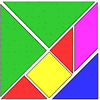### Tangrams

Can you make five differently sized squares from the interactive tangram pieces?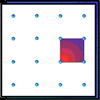### Geoboards

This practical challenge invites you to investigate the different squares you can make on a square geoboard or pegboard.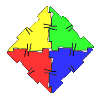### Polydron

This activity investigates how you might make squares and pentominoes from Polydron.

# Three Squares

##### Age 5 to 11Challenge Level
We had quite a lot of solutions come in for this task. I've shown here the ones who have seen that overlapping in a diagonal way produces the most squares. Other arrangements were not able to find nearly as many squares.

Children from St Joseph's Corinda Australia sent in their solutions

We quickly figured out that the squares won't work horizontally or vertically, so we place them diagonally. By touching the first two squares, and overlapping the third square in the middle, we had three big squares and four small squares in the middle for a total of seven squares. We then realised that if we overlapped the first two squares, we would create an extra square in the middle for a total of eight.

For four squares, we continued with overlapping diagonally. We added a square at the bottom
and managed to get a total of fifteen squares.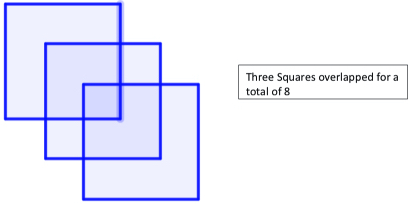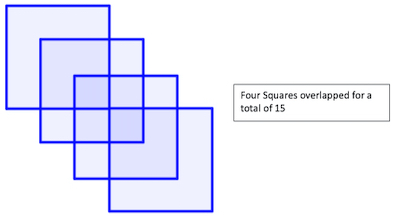Mrs. Harrell's Crew from Glenolden School in the U.S. wrote:

We realized from the example that we would have to overlap even more to try to get more than just five squares.
We tried to first overlap all three squares with at least one corner in common. At first, we were excited to have gotten what we thought was six squares, but then we noticed there were more than just three big squares and three tiny squares, there were also two medium squares.Andy from the American International School of Guangzhou (AISG) in China said:

In this problem, we had to make the most squares as it is possible. I made eight squares using three squares and using four squares I made 13 squares.
You play this game like you have to overlap the squares and make many squares. My method was to keep trying different shapes with squares.
I got eight squares by putting two square edge to edge and put the last one in the middle.
I got 13 squares by same as how I got eight squares but I put one more in the bottom.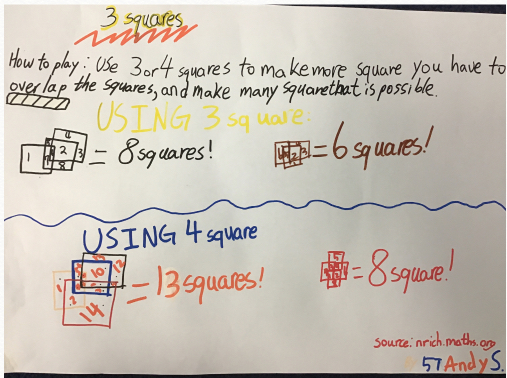Sara also from the American International School Of Guangzhou (AISG) said:

For this problem, I was able to make seven squares using three squares and 13 using four squares. First, using three squares I placed one square in the middle and placed another on top with a rectangle in the middle of the two squares. By doing this, I was able to split the rectangle in two creating two extra squares. Also, by placing the third square in the middle, I was able to create another two extra squares. Adding the next three squares originally, creating seven squares. (See picture below.)
Using four squares, I made 13 squares. I found two different ways to overlap the squares but still ended up with 13 squares. I placed, again, a square in the middle and placed two on the top left and right side of the first square. And, on top of that the last of the four squares.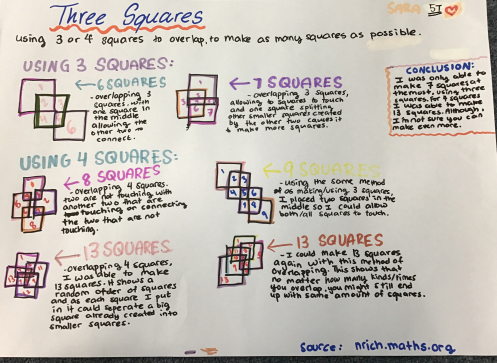Abbots Langley School Maths Club sent in their thoughts:

We think that the highest number of squares is eight.
To work this out we overlapped the squares as many times as we could. We realised that in order to get the highest number of squares we would need to overlap every square with the result being a square.

Well done everyone, although if anyone has any further thoughts about four squares overlapping, then please get in touch!